Back to Nautical Solar Sys. Hour Angles PZX Triangles Dip Amplitude Day Ex Meridian Exercises Pole Star Naut. Almanac

PZX Triangles

The apparent position of a body in the sky is defined by the horizon system of coordinates. In this system, the observer is located at the center of a imaginary hollow sphere of infinite diameter, the celestial sphere, which is divided into two hemispheres by the plane of the celestial horizon.

The altitude, H, is the vertical angle between the line of sight to the respective body and the celestial horizon, measured from 0° through +90° when the body is above the horizon (visible) and from 0° through -90° when the body is below the horizon (invisible).

The zenith distance, z, is the corresponding angular distance between the body and the zenith, an imaginary point vertically overhead of the observer. The zenith distance is measured from 0° through 180°. The point opposite to the zenith is called nadir (z = 180°).

H and z are complementary angles (H + z = 90°).

The azimuth, AzN, is the horizontal direction of the body with respect to the geographic (true) north point on the horizon, measured clockwise from 0° through 360°.

The Bearing Amplitude of a body is the arc of the horizon contained between the East point and the object when rising or between the West point and the object when setting.

It is defined as the angle at the zenith between the prime vertical and the vertical circle of the body when on the horizon.

A body always rises and sets on a Northerly bearing when its declination is North and on a Southerly bearing when its declination is South,

Only when the declination of the body is 0˚ does it rises in the East and sets in the West.

The amplitude thus takes the same name as the declination.

The amplitude at rising or setting of the body is the complement of the Azimuth, thus,

Azimuth = (90˚ - Amplitude)

Since Refraction is the greatest when the body is low on the horizon, altitude is 0˚; therefore the body appears about 33’ above its true position. Or we may say that the body is seen to rise before it actually does so.

The time of sunrise is when the sun is on the observer’s rational horizon, the amplitude of the sun therefore should be taken when the centre of the sun is about 33’ above the visible horizon.

This would mean that the time to take would be when the sun’s Lower Limb is half the semi diameter above the visible horizon.

Twilight

Dawn, Dusk or twilight is formed when the sun’s indirect rays appear above the horizon due to the refraction and reflection of the sun’s rays in the atmosphere.

Astronomical twilight begins when the sun is 18˚ below the horizon.

Nautical twilight begins when the sun is 12˚ below the horizon and Civil twilight begins when the sun is 6˚ below the horizon.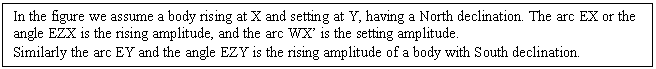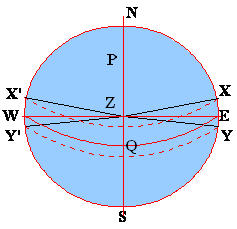Continuous twilight is experienced at latitude where the latitude:

Latitude + declination + 18˚ = 90˚

This zone would extend to a latitude of 18˚ above the latitude as obtained above.

Example:

Sun’s Declination 22˚N

Twilight would be in Latitudes:

Latitude + declination + 18˚ = 90˚

Latitude + 22 + 18˚ = 90˚

Latitude = 50˚

And the zone would be 50 + 18 = 68

So the latitude within the belt of 50˚ to 68˚ would experience Twilight and total darkness would not be there.

Again from the above:

Polar Distance = 90˚ - declination

Or P =  90 – 22

P = 68

Therefore the sun would become circumpolar above 68˚N and there would be daylight 24hours of the day.

Twilight is shorter closer to the equator than in higher latitudes since the sun rises and sets nearly perpendicular to the horizon and thus passes through the above 18˚ belt very quickly, unlike in the higher latitudes where the sun rises and sets obliquely and thus takes a longer time to cross the 18˚ belt.

Circumpolar and the conditions necessary for a body to be circumpolar

All celestial bodies are circumpolar, that is they describe a circle around a pole. However when a body is circumpolar we mean that the body is visible at their lower transit across the meridian.

A celestial body being on the same hemisphere as the observer is either sometimes above the horizon or circumpolar. A body being on the opposite hemisphere is either sometimes above the horizon or permanently invisible, but never circumpolar.

The sun provides a good example of how the visibility of a body is affected by latitude and declination. At the time of the summer solstice (Dec = +23.5°), the sun is circumpolar to an observer being north of the Arctic Circle (Lat > +66.5°). At the same time, the sun remains below the horizon all day if the observer is south of the antarctic circle (Lat < 66.5°). At the times of the equinoxes (Dec = 0°), sun is circumpolar only at the poles. At the time of the winter solstice (Dec = (-) 23.5°), the sun is circumpolar south of the Antarctic Circle and invisible north of the Arctic Circle. If the observer is between the arctic and the Antarctic Circle, the sun is visible during a part of the day all year round.

Assume that an observer is at Latitude of 50°N, he observes a body whose declination is 56°N.

Thus the polar distance would be (90-56 = 34°)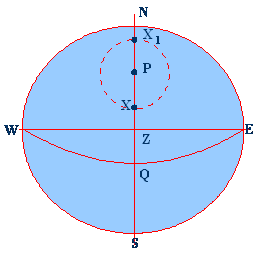The pole P is not at the centre of the diurnal arc of the body, unless however the body is quite close to the pole.

The upper meridian passage is at X, when the body is between the pole and the zenith. And the lower meridian passage is at X1, when the body is between the pole and the horizon.

The Altitudes are 16° when below the pole (NX1) and 84° when above the pole (NX)

PX = PX1 are the polar distances.

The latitude may be found by observing the altitudes of any circumpolar body while it is above the meridian and again while it is below the meridian.

Lat = ½ (upper meridian altitude + lower meridian altitude)

In the above case:

Lat = ½ (16°+84°) = 50°

From the above figure we see that:

Lat = ½ (NX + NX1)

Lat = ½ (NP + PX + NP – PX1) = ½ (2NP)

Or Lat = NP

A body is circumpolar (always above the celestial horizon) if the zenith distance is smaller than 90° at the moment of lower meridian passage. The following conditions should exist:

A body is continually below the celestial horizon if the zenith distance is greater than 90° at the instant of upper meridian passage.

The parts of the PZX triangle

To understand the PZX triangle, the concept of the celestial and the terrestrial spheres should be clear.

For an observer looking to the heavens, the heavenly bodies are imagined to be equidistant from him and are fixed for the moment of the observation on a giant sphere, which has as its centre coinciding with the centre of the earth.

Thus for the observer he is standing on a sphere (terrestrial) and looking outward at the bodies painted on the inner surface of another sphere (celestial)

The bodies on the celestial sphere if dropped onto the surface of the earth would remain at the same GP.

Thus the two spheres are geocentric. And all the imaginary divisional lines on the earth have corresponding celestial equivalents.

There is a major difference; unlike on the surface of the earth all distances on the celestial sphere are angular distances.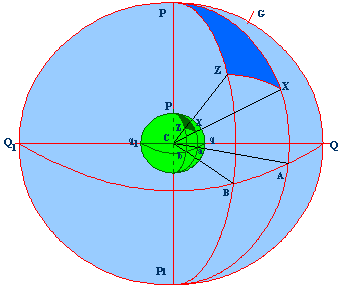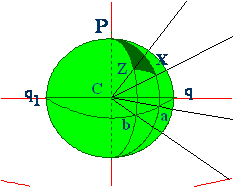The positions of the points PZX on the celestial sphere correspond to the point’s pzx on the earth’s surface.

Thus the virtual positions on the celestial sphere have their definite positions on the earth’s surface.

Declination – of a body is the arc of the celestial meridian contained between the equinoctial and the body. Thus the declination of point X is the arc AX, this corresponds to the arc ax on the surface of the earth, since both the arcs subtend the same angle ACX so the declination of

X = arc AX = arc ax

Polar Distance (PX, is the arc of a celestial meridian between the body and the elevated pole. PX represents the polar distance of X, and this arc corresponds to px on the earth as both arcs subtend the same angle PCX, so that the polar distance of

X – arc PX = arc px

The elevated pole is the one above the horizon. The North Pole is the elevated pole for an observer in North Latitude, and South Pole being below the horizon would be the depressed pole.

The Zenith  is the spot vertically over the observer. Suppose z in the figure to be the position of an observer on the earths surface, then cz produced would project the spot Z on the celestial sphere.

But bz represents the latitude of z on the earth and this arc corresponds to the arc BZ on the celestial sphere as both subtend the same angle BCZ at the centre of the earth, so that the

Latitude of z = arc bz = arc BZ

Similarly arc pz is equal to the celestial arc PZ as both subtend the same angle PCZ, this is the co-latitude of z and is equal 90° - Latitude = arc pz = arc PZ

Again if ZX be the arc of the celestial great circle joining the points Z and Z, then the arc zx is the terrestrial gre4at circle joining the corresponding points on the earths surface.

The arcs ZX and zx are both equal to angle ZCX, the arc ZX measures the angular distance of the body X from the zenith and the arc zx measures not only the angular distance between x and the observer, but also the great circle distance in miles between them.

Thus we see that the celestial PZX points are projected on the earth’s surface as pzx. These three points make up the triangle PZX and is known as the Pole – Zenith – Star triangle.

The three sides of the triangle are thus:

PZ = pz = co – latitude = 90° - Lat. And is obtained from the ships DR position

PX = px = polar distance = co – decl = 90° - decl., obtained from the Nautical Almanac

ZX = zx = zenith distance = co – altitude = 90° - alt., obtained from the sextant.

The Zenith Distance ZX, is the angular distance of the body from the zenith.

True Altitude is the angular height of the centre of the body above the observer’s rational horizon. This arc in the first instance is measured with a sextant and the altitude so obtained is reduced to what it would be if the body were observed from the centre of the earth instead of from the surface.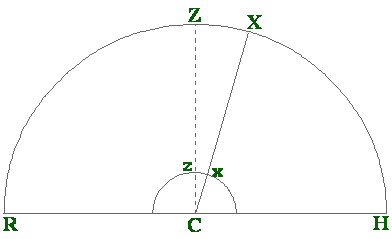Above RCH represents the plane of the rational horizon. This plane passes through the centre of the earth (the small semi circle).

The observer is on the surface of the earth at z. The point Z on the celestial sphere is the projection of the top of the observer. The star is at point X on the celestial sphere, and the GP of the star X is on the surface of the earth at x.

The arc HX (angle HCX) is the true altitude of the star X.

Then ZX would be the zenith distance – ZH – HX or 90° - altitude.

The arc ZX corresponds to the arc zx on the earth’s surface or angle ZCX at the centre of the earth.

In spherical trigonometry unlike in normal trigonometry, the sides of a triangle are expressed in degrees.

In plane triangle the sides of a triangle may not be equal, although the angles subtended by the sides may be equal.In spherical trigonometry however since the sides are measured as arc that is in degrees the sides of a larger triangle and that of a smaller triangle are equal being the fact that both have the same arc measurement.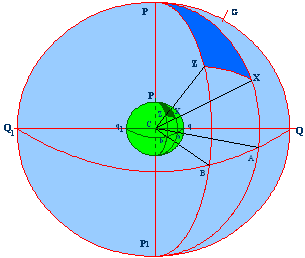In the above figure the angle P in the triangle PZX is the most important and it represents the HOUR ANGLE of the star X

LOCAL HOUR ANGLE of a body is the angle at the pole contained between the meridian of the observer (PZ) and the meridian of the body (PX), that is the angle ZPX that is the same angular measure as the arc AB.